×#### Thank you for registering.

One of our academic counsellors will contact you within 1 working day.

Click to Chat

1800-1023-196

+91-120-4616500

CART 0

• 0

MY CART (5)

Use Coupon: CART20 and get 20% off on all online Study Material

ITEM
DETAILS
MRP
DISCOUNT
FINAL PRICE
Total Price: Rs.

There are no items in this cart.
Continue Shopping• Complete JEE Main/Advanced Course and Test Series
• OFFERED PRICE: Rs. 15,900
• View Details

```Chapter 8: Quadratic Equations Exercise – 8.13

Question: 1

Find the roots of the equation (x - 4) (x + 2) = 0

Solution:

The given equation is (x - 4)(x + 2) = 0

Either x - 4 = 0 therefore x = 4

Or, x + 2 = 0 therefore x = -2

The roots of the above mentioned quadratic equation are 4 and -2 respectively.

Question: 2

Find the roots of the equation (2x + 3)(3x - 7) = 0

Solution:

The given equation is (2x + 3)(3x - 7) = 0.

Either 2x + 3 = 0, therefore x = (-3)/2

Or, 3x -7 = 0, therefore x = 7/3

The roots of the above mentioned quadratic equation are x = (-3)/2 and x =7/3 respectively.

Question: 3

Find the roots of the quadratic equation 3x2 - 14x - 5 = 0

Solution:

The given equation is 3x2 - 14x - 5 = 0

= 3x2 - 14x - 5 = 0

= 3x2 - 15x + x - 5 = 0

= 3x(x - 5) + 1(x - 5) = 0

= (3x + 1)(x - 5) = 0

Either 3x + 1 = 0 therefore x = (-1)/3

Or, x - 5 = 0 therefore x = 5

The roots of the given quadratic equation are 5 and x = (-1)/3 respectively.

Question: 4

Find the roots of the equation 9x2 - 3x - 2 = 0.

Solution:

The given equation is 9x2 - 3x - 2 = 0.

= 9x2 - 3x - 2 = 0.

= 9x2 - 6x + 3x - 2 = 0

= 3x(3x - 2) + 1(3x - 2) = 0

= (3x - 2)(3x + 1) = 0

Either, 3x - 2 = 0 therefore x = 2/3

Or, 3x + 1 = 0 therefore x = (-1)/3

The roots of the above mentioned quadratic equation are x = 2/3 and x = (-1)/3 respectively.

Question: 5

Find the roots of the quadratic equation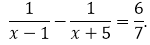Solution:

The given equation is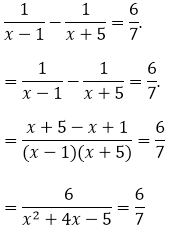Cancelling out the like terms on both the sides of the numerator. We get,= x2 + 4x - 5 = 7

= x2 + 4x - 12 = 0

= x2 + 6x - 2x - 12 = 0

= x(x + 6) - 2(x - 6) = 0

= (x + 6)(x - 2) = 0

Either x + 6 = 0

Therefore x = -6

Or, x - 2 = 0

Therefore x = 2

The roots of the above mentioned quadratic equation are 2 and – 6 respectively.

Question: 6

Find the roots of the equation 6x2 + 11x + 3 = 0.

Solution:

The given equation is 6x2 + 11x + 3 = 0.

= 6x2 + 11x + 3 = 0.

= 6x2 + 9x + 2x + 3 = 0

= 3x(2x + 3) + 1(2x + 3) = 0

= (2x + 3)(3x + 1) = 0

Either, 2x + 3 = 0 therefore x = (-3)/2

Or, 3x + 1 = 0 therefore x = (-1)/3

The roots of the above mentioned quadratic equation are x = (– 3)/2 and x = (– 1)/2 respectively.

Question: 7

Find the roots of the equation 5x2 - 3x - 2 = 0

Solution:

The given equation is 5x2 - 3x - 2 = 0.

= 5x2 - 3x - 2 = 0.

= 5x2 - 5x + 2x - 2 = 0

= 5x(x - 1) + 2(x - 1) = 0

= (5x + 2)(x - 1) = 0

Either 5x + 2 = 0 therefore x = (- 2)/5

Or, x - 1 = 0 therefore x = 1

The roots of the above mentioned quadratic equation are 1 and x = (- 2)/5 respectively.

Question: 8

Find the roots of the equation 48x2 - 13x - 1 = 0

Solution:

The given equation is 48x2 - 13x - 1 = 0.

= 48x2 - 13x - 1 = 0.

= 48x2 - 16x + 3x - 1 = 0.

= 16x(3x - 1) + 1(3x - 1) = 0

= (16x + 1)(3x - 1) = 0

Either 16x + 1 = 0 therefore (-1)/16

Or, 3x - 1 = 0 therefore x = 1/3

The roots of the above mentioned quadratic equation are x = (-1)/16 and x = 1/3 respectively.

Question: 9

Find the roots of the equation 3x2 = – 11x – 10

Solution:

The given equation is 3x2 = – 11x – 10

= 3x2 = – 11x - 10

= 3x2 + 11x + 10 = 0

= 3x2 + 6x + 5x + 10 = 0

= 3x(x + 2) + 5(x + 2) = 0

= (3x + 2)(x + 2) = 0

Either 3x + 2 = 0 therefore x = (- 2)/3

Or, x + 2 = 0 therefore x = -2

The roots of the above mentioned quadratic equation are (-2)/3 and – 2 respectively.

Question: 10

Find the roots of the equation 25x(x + 1) = – 4

Solution:

The given equation is 25x(x + 1) = 4

= 25x(x + 1) = – 4

= 25x2 + 25x + 4 = 0

= 25x2 + 20x + 5x + 4 = 0

= 5x(5x + 4) + 1(5x + 4) = 0

= (5x + 4)(5x + 1) = 0

Either 5x + 4 = 0 therefore x = (- 4)/5

Or, 5x + 1 = 0 therefore x = (- 1)/5

The roots of the quadratic equation are x = (- 4)/5 and x = (- 1)/5 respectively.

Question: 11

Find the roots of the quadratic equation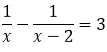Solution:

The given equation isCross multiplying both the sides. We get,

= 2 = 3x(x - 2)

= 2 = 3x2 - 6x

= 3x2 - 6x - 2 = 0

= 3x2 - 3x - 3x - 2 = 0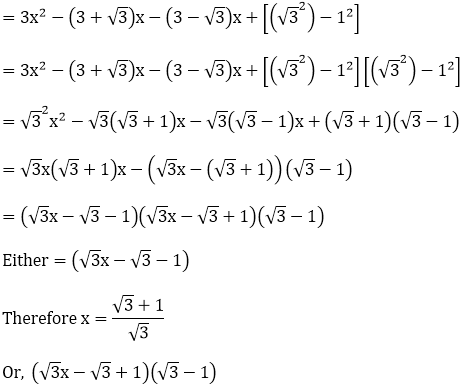The roots of the above mentioned quadratic equation areand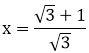respectively.

Question: 12

Find the roots of the quadratic equation x − 1/x = 3

Solution:

The given equation is x − 1/x = 3= x2 - 1 = 3x

= x2 - 1 - 3x = 0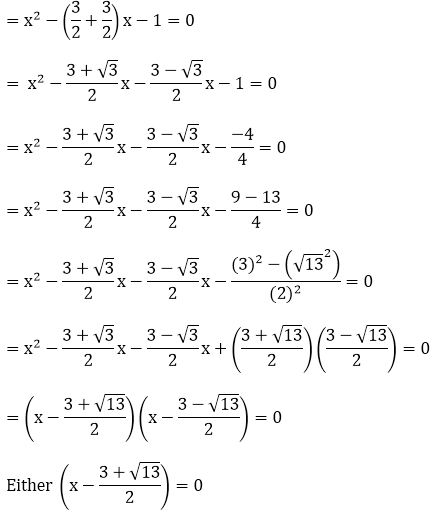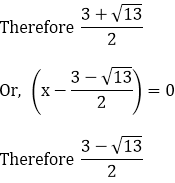The roots of the above mentioned quadratic equation areandrespectively.

Question: 13

Find the roots of the quadratic equation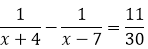Solution:

The given equation isCancelling out the like numbers on both the sides of the equation= x2 - 3x - 28 = -30

= x2 - 3x - 2 = 0

= x2 - 2x - x - 2 = 0

= x(x - 2) - 1(x - 2) = 0

= (x - 2)(x - 1) = 0

Either x - 2 = 0

Therefore x = 2

Or, x - 1 = 0

Therefore x = 1

The roots of the above mentioned quadratic equation are 1 and 2 respectively.

Question: 14

Find the roots of the quadratic equation a2x2 - 3abx + 2b2 = 0

Solution:

The given equation is a2x2 - 3abx + 2b2 = 0

= a2x2 - 3abx + 2b2 = 0

= a2x2 - abx - 2abx + 2b2 = 0

= ax(ax - b) - 2b(ax - b) = 0

= (ax - b)(ax - 2b) = 0

Either ax - b = 0 therefore x = b/a

Or, ax - 2b = 0 therefore x = 2b/a

The roots of the quadratic equation are x = 2b/a and x = b/a respectively.

Question: 15

Find the roots of the 4x2 + 4bx - (a2 - b2) = 0

Solution:

= - 4(a2 - b2) = - 4(a - b)(a + b)

= -2(a - b) * 2(a + b)

= 2(b - a) * 2(b + a)

= 4x2 + (2(b - a) + 2(b + a)) – (a - b)(a + b) = 0

= 4x2 + 2(b - a)x++ 2(b + a)x + (b - a)(a + b) = 0

= 2x(2x + (b - a)) + (a + b)(2x + (b - a)) = 0

= (2x + (b - a))(2x + b + a) = 0

Either, (2x + (b - a)) = 0Or, (2x + b + a) = 0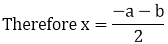The roots of the above mentioned quadratic equation are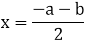and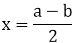respectively.

Question: 16

Find the roots of the equation ax2 + (4a2 - 3b)x - 12ab = 0

Solution:

The given equation is ax2 + (4a2 - 3b)x - 12ab = 0

= ax2 + (4a2 - 3b)x - 12ab = 0

= ax2 + 4a2x - 3bx -12ab = 0

= ax(x - 4a) – 3b(x - 4a) = 0

= (x - 4a)(ax - 4b) = 0

Either x - 4a = 0

Therefore x = 4a

Or, ax - 4b = 0

Therefore x = 4b/a

The roots of the above mentioned quadratic equation are x = 4b/a and 4a respectively.

Question: 17

Find the roots ofSolution:

The given equation is= (x + 3)(2x - 3) = (x + 2)(3x - 7)

= 2x2 - 3x + 6x - 9 = 3x2 - x - 14

= 2x2 + 3x - 9 = 3x2 - x - 14

= x2 - 3x - x - 14 + 9 = 0

= x2 - 5x + x - 5 = 0

= x(x - 5) + 1(x - 5) = 0

= (x - 5)(x + l) - 0

Either x - 5 - 0 or x + 1= 0

x = 5 and x = –1

The roots of the above mentioned quadratic equation are 5 and -1 respectively.

Question: 18

Find the roots of the equation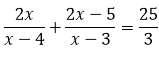Solution:

The given equation is= 3(4x2 - 19x + 20) = 25(x2 - 7x + 12)

= 12x2 - 57x + 60 = 25x2 – 175x + 300

= 13x2 - 78x - 40x + 240 = 0

= 13x2 - 118x + 240 = 0

= 13x2 - 78x - 40x + 240 = 0

= 13x(x - 6) - 40(x - 6) = 0

= (x - 6)(13x - 40) = 0

Either x - 6 = 0 therefore x = 6

Or , 13x - 40 = 0 therefore x = 40/13

The roots of the above mentioned quadratic equation are 6 and 40/13 respectively.

Question: 19

Find the roots of the quadratic equation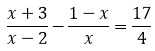Solution:

The given equation is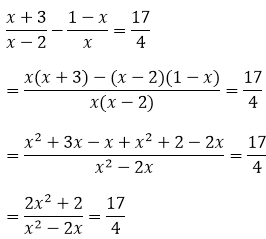= 4(2x2 + 2) = 17(x2 - 2x)

= 8x2 + 8 = 17x2 - 34x

= 9x2 - 34x - 8 = 0

= 9x2 - 36x + 2x - 8 = 0

= 9x(x - 4) + 2(x - 4) = 0

= (9x + 2)(x - 4) = 0

Either 9x + 2 = 0 therefore x = (-2)/9

Or, x - 4 = 0 therefore x = 4

The roots of the above mentioned quadratic equation are x = (-2)/9 and 4 respectively.

Question: 20

Find the roots of the quadratic equation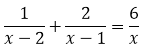Solution:

The equation is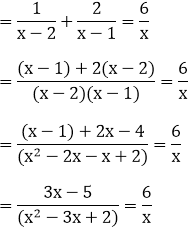= x(3x - 5) = 6(x2 - 3x + 2)

= 3x2 - 5x = 6x2 - 18x + 12

= 3x2 - 13x + 12 = 0

= 3x2 - 9x - 4x + 12 = 0

= 3x(x - 3) - 4(x - 3) = 0

= (x - 3)(3x - 4) = 0

Either x - 3 = 0 therefore x = 3

Or, 3x - 4 = 0 therefore 4/3

The roots of the above mentioned quadratic equation are 3 and 4/3 respectively.

Question: 21

Find the roots of the quadratic equation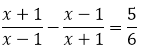Solution:

The equation is= 6(4x) = 5(x2 - 1)

= 24x = 5x2 - 5

= 5x2 - 24x - 5 = 0

= 5x2 - 25x + x - 5 = 0

= 5x(x - 5) + 1(x - 5) = 0

= (5x + 1)(x - 5) = 0

Either x - 5 = 0

Therefore x = 5

Or, 5x + 1 = 0

Therefore x = (-1)/5

The roots of the above mentioned quadratic equation are x = (-1)/5 and 5 respectively.

Question: 22

Find the roots of the quadratic equationSolution:

The equation is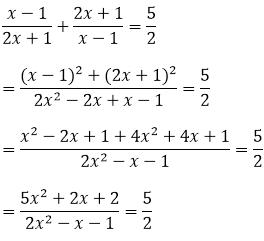= 2(5x2 + 2x + 2) = 5(2x2 - x - 1)

= 10x2 + 4x + 4 = 10x2 - 5x - 5

Cancelling out the equal terms on both sides of the equation. We get,

= 4x + 5x + 4 + 5 = 0

= 9x + 9 = 0

= 9x = – 9

x = –1

x = – 1 is the only root of the given equation.

Question: 23

Find the roots of the quadratic equation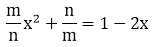Solution:

The given equation isNow we solve the above quadratic equation using factorization method

Therefore,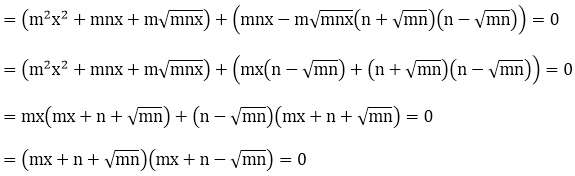Now, one of the products must be equal to zero for the whole product to be zero for the whole product to be zero. Hence, we equate both the products to zero in order to find the value of x.

Therefore,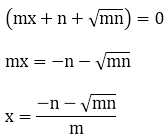Or,The roots of the above mentioned quadratic equation are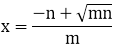andrespectively.

Question: 24

Find the roots of the quadratic equationSolution:

The given equation is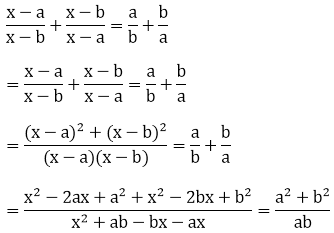= (2x2 - 2x(a + b) + a2 + b2)ab = (a2 + b2)(x2 - (a + b)x + ab)

= (2abx2 - 2abx(a + b) + ab(a2 + b2)) = (a2 + b2)(x2 - (a2 + b2)(a + b)x + (a2 + b2)(ab)

= (a2 + b2 - 2ab)x - (a + b)(a2 + b2 - 2ab)x = 0

= (a - b)2x2 - (a + b)(a + b)2x2 = 0

= x(a - b)2(x - (a + b)) = 0

= x(x - (a + b)) = 0

Either x = 0

Or, (x - (a + b)) = 0

Therefore x = a + b

The roots of the above mentioned quadratic equation are 0 and a + b respectively.

Question: 25

Find the roots of the quadratic equationSolution:

The given equation is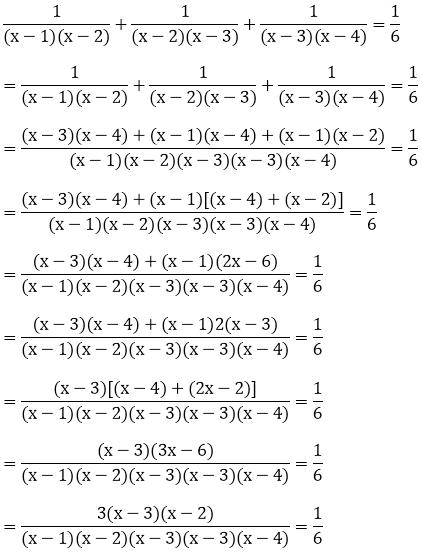Cancelling out the like terms on both the sides of numerator and denominator. We get,= (x - 1)(x - 4) = 18

= x2 - 4x - x + 4 = 18

= x2 - 5x - 14 = 0

= x2 - 7x + 2x - 14 = 0

= x(x - 7) + 2(x - 7) = 0

= (x - 7)(x + 2) = 0

Either x - 7 = 0

Therefore x = 7

Or, x + 2 = 0

Therefore x = – 2

The roots of the above mentioned quadratic equation are 7 and – 2 respectively.

Question: 26

Find the roots of the quadratic equation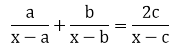Solution:

The given equation is= (x - c)(ax - 2ab + bx) = 2c(x2 - bx - ax + ab)

= (a + b)x2 - 2abx - (a + b)cx + 2abc = 2cx2 - 2c(a + b)x + 2abc

Question: 27

Find the roots of the Question x2 + 2ab = (2a + b)x

Solution:

The given equation is x2 + 2ab = (2a + b)x

= x2 + 2ab = (2a + b)x

= x2 - (2a + b)x + 2ab = 0

= x2 - 2ax - bx + 2ab = 0

= x(x - 2a) - b(x - 2a) = 0

= (x - 2a)(x - b) = 0

Either x - 2a = 0

Therefore x = 2a

Or, x - b = 0

Therefore x = b

The roots of the above mentioned quadratic equation are 2a and b respectively.

Question: 28

Find the roots of the quadratic equation (a + b)2x2 - 4abx - (a - b)2 =0

Solution:

The given equation is (a + b)2x2 - 4abx - (a - b)2 = 0

= (a + b)2x2 - 4abx - (a - b)2 = 0

= (a + b)2x2 - ((a + b)2 - (a-b)2 )x - (a - b)2  = 0

= (a + b)2x2 - (a + b)2 x + (a - b)2 x - (a - b)2 = 0

= (a + b)2x(x - 1) (a + b)2(x - 1) = 0

= (x - 1)(a + b)2x + (a + b)2) = 0

Either x - 1 = 0

Therefore x = 1

Or, (a + b)2x + (a + b)2) = 0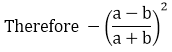The roots of the above mentioned quadratic equation are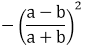and 1 respectively.

Question: 29

Find the roots of the quadratic equation a(x2 + 1) - x(a2 + 1) = 0

Solution:

= a(x2 + 1) - x(a2 + 1) = 0

= ax2 + a - a2x - x = 0

= ax(x - a) - 1(x - a) = 0

= (x - a)(ax - 1) = 0

Either x - a = 0

Therefore x = a

Or, ax - 1 = 0

Therefore x = 1/a

The roots of the above mentioned quadratic equation are (a and x = 1/a respectively.

Question: 30

Find the roots of the quadratic equationSolution:

The given equation is= x2 + (a + 1/a)x + 1 = 0

= x2 + ax + x/a +(a × 1/a) = 0

= x(x + a) +1/a(x + a) = 0

= (x + a)(x + 1/a) = 0

Either x + a = 0

Therefore x = –  a

Or , (x + 1/a) = 0

Therefore x = 1/a

The roots of the above mentioned quadratic equation are x = 1/a and a respectively.

Question: 31

Find the roots of the quadratic equation abx2 + (b2 - ac)x - bc = 0

Solution:

The given equation is abx2 + (b2 - ac)x - bc = 0

= abx2 + (b2 - ac)x - bc = 0

= abx2 + b2x - acx - bc = 0

= bx(ax + b) - c(ax + b) = 0

= (ax + b)(bx - c) = 0

Either, ax + b = 0

Therefore x = (-b)/a

Or, bx - c = 0

Therefore x = c/b

The roots of the above mentioned quadratic equation are x = c/b and x = (-b)/a respectively.

Question: 32

Find the roots of the quadratic equation a2b2x2 + b2x - a2x - 1 = 0

Solution:

The given equation is a2b2x2 + b2x - a2x - 1 = 0

= a2b2x2 + b2x-a2x - 1 = 0

= b2x(a2x + 1) - 1(a2x + 1)

= (a2x + 1)( b2x - 1) = 0

Either (a2x + 1) = 0

Therefore x = (-1)/a2

Or, (b2x - 1) = 0

Therefore x = 1/b2

The roots of the above mentioned quadratic equation are x = 1/b2 and x = (-1)/a2 respectively.
```### Course Features

• 728 Video Lectures
• Revision Notes
• Previous Year Papers
• Mind Map
• Study Planner
• NCERT Solutions
• Discussion Forum
• Test paper with Video Solution